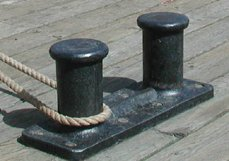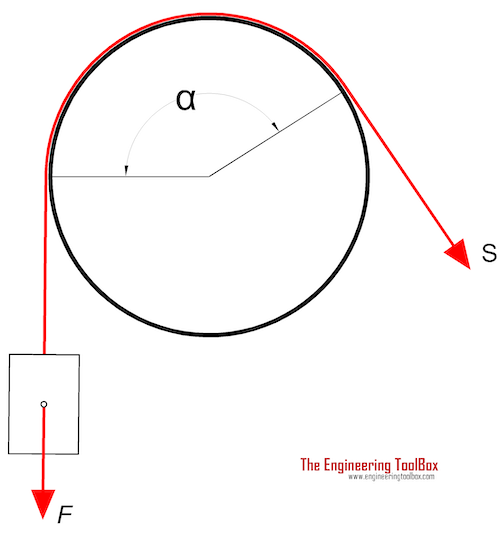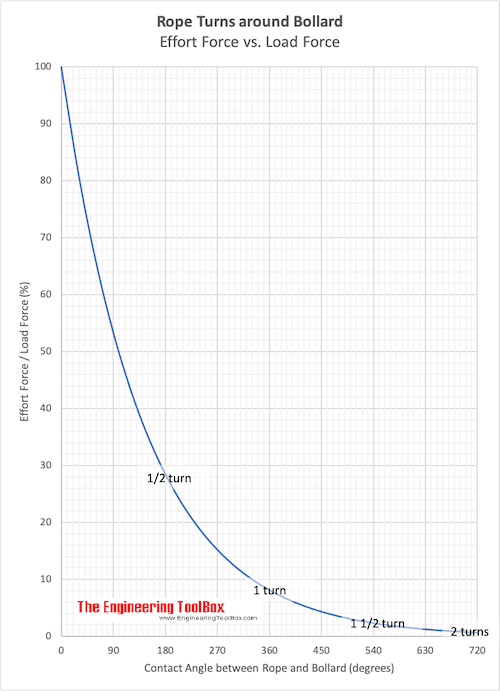Engineering ToolBox - Resources, Tools and Basic Information for Engineering and Design of Technical Applications!

# Bollard Forces

## Friction, load and effort forces acting in ropes turned around bollards.Bollards are common on quays and used to moore ships and boats. By turning a rope around a pole the effort force required to hold the load force can be reduced dramatically.The effort force in a rope around a bollard can be calculated as

S = F e-(μ αrad)                         (1)

where

S = effort force in the rope (N, lb)

F = load force (N, lbf)

e = 2.718..

μ = friction coefficient (approximately 0.3 - 0.5 is common for a rope around a steel or cast iron bollard)

αrad = 2 π αdegrees / 360 = angle where the rope is in contact with the bollard (radians

αdegrees = angle where the rope is in contact with the bollard (degrees)

### Angle - turns, degrees and radians

• 1/4 turn : 90 degrees => α = 1/2 π
• 1/2 turn : 180 degrees => α = π
• 1 turn : 360 degrees => α = 2 π
• 2 turns : 720 degrees => α = 4 π

### Example - A rope with One turn around a Bollard

One turn equals to 360 degrees or 2 π radians. With a friction coefficient of 0.5 the effort force in the rope can be calculated as

S = F e-(0.5 2 π)

= 0.043 F (N)

One turn around the bollard reduces the required effort force to less than 5% of the load force.

### Example - Shoring a ship

The retardation (negative acceleration) of a ship arriving at quay with velocity 0.05 m/s and stopped within 2 seconds - can be calculated as

a = dv/dt                                (2)

= (0.05 m/s) / (2 s)

= 0.025 (m/s2)

With ship mass 20000 kg the required force in the rope from the ship (load) can be calculated as

F = m a                                      (3)

= (20000 kg) (0.025 m/s2)

= 500 N

= 0.5 kN

The required effort force in the rope with a half turn around the bollard (180 degrees or π radians) with a friction coefficient of 0.4 can be calculated as

S = (500 N) e-0.4 π

= 142 N

= 0.14 kN

The Effort Force - Load Force ratio for various rope angles are indicated in the chart below:• friction coefficient 0.5

### Bollard (or Pole) Effort Force Calculator

This calculator is based on the equation above and can be used to calculate the effort force in ropes turned around poles or bollards. Note that the calculator uses degrees for the turns.

friction coefficient

angle where the rope is in contact with the pole (degrees)

## Related Topics

• ### Dynamics

Motion - velocity and acceleration, forces and torque.
• ### Mechanics

Forces, acceleration, displacement, vectors, motion, momentum, energy of objects and more.
• ### Statics

Loads - forces and torque, beams and columns.

## Related Documents

• ### Equilibrant Force

The force required to keep a system of forces in equilibrium.
• ### Force

Newton's third law - force vs. mass and acceleration.
• ### Forces and Tensions in Ropes due to Angle

Reduced load capacities in ropes, cables or lines - due to acting angle.
• ### Friction - Friction Coefficients and Calculator

Friction theory with calculator and friction coefficients for combinations of materials like ice, aluminum, steel, graphite and many more.
• ### Impact Force

Impact forces acting on falling objects hitting the ground, cars crashing and similar cases.
• ### Manila Ropes - Strength

Minimum breaking strength and safe load of manila 3-strand rope.
• ### Mass vs. Weight

Mass vs. weight - the Gravity Force.
• ### Nylon Ropes - Strengths

Nylon 3-strand and 8-strand rope - minimum breaking strength and safe load.
• ### Polyester Polyolefin Ropes - Strengths

Polyester Polyolefin dual fiber 3-strand rope - minimum breaking strength and safe load.
• ### Polyester Ropes - Strengths

Polyester 3-strand and 8-strand rope - minimum breaking strength and safe load.
• ### Polypropylene Fiber Ropes - Strengths

Polypropylene fiber 3-strand and 8 strand rope - minimum breaking strength and safe load.

• ### Shipping Measuring Units

Vessel and cargo capacities.

Online vector calculator - add vectors with different magnitude and direction - like forces, velocities and more.
• ### Wire Rope Slings

Sling angles and influence on capacity.

## Engineering ToolBox - SketchUp Extension - Online 3D modeling!

Add standard and customized parametric components - like flange beams, lumbers, piping, stairs and more - to your Sketchup model with the Engineering ToolBox - SketchUp Extension - enabled for use with older versions of the amazing SketchUp Make and the newer "up to date" SketchUp Pro . Add the Engineering ToolBox extension to your SketchUp Make/Pro from the Extension Warehouse !

We don't collect information from our users. More about

## Citation

• The Engineering ToolBox (2008). Bollard Forces. [online] Available at: https://www.engineeringtoolbox.com/bollard-force-d_1296.html [Accessed Day Month Year].

Modify the access date according your visit.

9.19.12